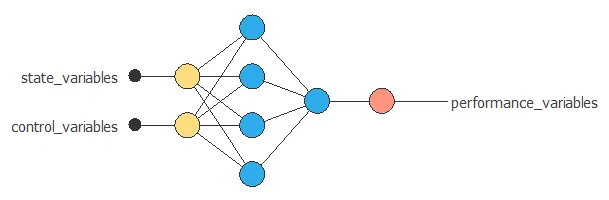Tracking the behavior of a system allows us to predict its behavior and improve its performance.

This article explains how machine learning can be used to model engineering systems and optimize industrial processes.

Contents:

## Data set

The data set contains measurements from our system or process.

It comprises the following types of variables:

### State variables

State variables are those inputs that determine the performance of the system and are not actionable by the company’s technicians.

Some examples of state variables are:

• The ambient temperature in a combined cycle power plant.
• The water salinity in a desalination plant.

### Control variables

Control variables are those inputs that determine the performance of the system and can be set by the company’s technicians.

Two examples of control variables are:

• The velocity of an aircraft.
• The combustion air flows in a furnace.

### Performance variables

Performance variables are the system’s outputs and depend on the state and the control variables.

Performance optimization can consider different targets:### PROCESS EFFICIENCY### ENERGY CONSUMPTION### GAS EMISSIONS## Mathematical model

The model of a process is a mathematical description that adequately predicts the response of the physical system to all anticipated inputs.

More specifically, it relates the performance variables with the state variables and the control variables.

$$performance\_variables = function(state\_variables, control\_variables)$$

Neural networks are algorithms used to fit multi-dimensional and non-linear functions from data sets.The inputs to the neural network include the state and control variables. The outuputs from the neural network are the predicted performance variables of the system for that scenario.

## Response optimization

The objective of the response optimization algorithm is to exploit the mathematical model to look for optimal operating conditions.

A performance optimization problem might be also specified by a set of constraints on the inputs and the outputs of the system.

More specifically, the objective of performance optimization is to determine the control signals that will cause a process to satisfy the physical constraints and at the same time minimize or maximize some performance criterion.In output optimization, constraints are imposed on the controls. The objective is then to optimize the outputs.

$$optimize \> outputs\\ controls \in admissible\_controls$$

Here the outputs can be miminized or maximized.

An example is to minimize the fuel consumption of an aircraft while maintaining the speed at a desired value.

## Conclusions

To conclude, machine learning is a powerful technique to predict the performance of engineering systems.

In turn, predictive models allow us to simulate different operating scenarios and adjust the control variables to improve efficiency.

Some examples of performance optimization are to improve process efficiency or to reduce energy consumption.

Neural Designer uses neural networks to model the behaviour of systems. It also contains response optimization algorithms to fine tune the control variables and optimize the performance.